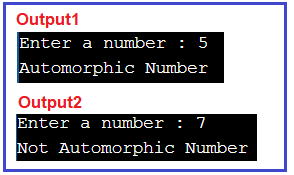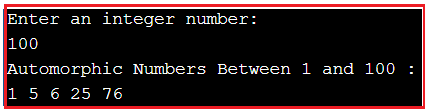# Automorphic Number in C#

## Automorphic Number Program in C# with Examples

In this article, I am going to discuss the How to Implement the Automorphic Number Program in C# with Examples. Please read our previous article where we discussed the Happy Number Program in C#. Here, in this article, first, we will learn what is a Automorphic Number and then we will see how to implement the Automorphic Number Program in C#. And finally, we will see how to print all the Automorphic numbers between a range of numbers like between 1 to 100 or 100 to 1000, etc.

##### What is an Automorphic Number?

A number is said to be an Automorphic number if the square of the number will contain the number itself at the end.
Example: 5 is an automorphic number
Explanation: 5 * 5 = 25 //ends with 5 which is the original number.

Example.: 7 is not an automorphic number
Explanation: 7 * 7 = 49 //doesn’t ends with the number 7

##### How to implement the Automorphic Number Program in C#?

We are going to use the very basic method to implement this program but before that, you should have some basic knowledge of the following operators.

1. Modulus(%) Operator
2. Divide(/) Operator

In case if you are unfamiliar or not confident about these let’s have a look at our previous questions where we have discussed these in great detail like Duck Number, Disarium Number, Buzz Number, and Strong Number, etc.

###### Solution

Step1: Take a number from the user.
Step2: Find the square of number by just multiplying it with the number itself and store this in a variable named square.
Step3: Calculate or extract the last digit of both (the square number and the given number) numbers using the modulus % operator.

Example: Given number: 25.
Square number: 625
25 % 10 = 5 625 % 10 = 5
2 % 10 = 2 62 % 10 = 2
0 % 10 =0 6 % 10 = 6
This process will continue until the remainder value is not equal to 0.

Step4: Compare the remainder of both the numbers. If it is not equal then immediately break the loop and print “Not Automorphic Number” otherwise repeat the process until the remainder =0 of the given number. Then simply Print the Automorphic Number.

Note: If the loop will continue till the end which means all the digits of the number are present in the squared number.

##### Example: Automorphic Number Program in C#

The following sample code shows how to implement the Automorphic number program in C#.

```using System;
public class AutomorphicProgram
{
public static void Main ()
{
Console.Write ("Enter a number : ");
int number = Convert.ToInt32 (Console.ReadLine ());

if (CheckAutomorphicNumber (number))
{
Console.WriteLine ("Automorphic Number");
}
else
{
Console.WriteLine ("Not Automorphic Number");
}

}

public static bool CheckAutomorphicNumber (int no)
{
int square = no * no;
while (no > 0)
{
if (no % 10 != square % 10)
{
return false;
}
no = no / 10;
square = square / 10;
}
return true;
}
}
```
###### Output##### C# Program to Print all Automorphic Numbers Between 1 and N

The following C# Program will allow the user to input a number. Then it will print all the Automorphic numbers between 1 and that input number.

```using System;
public class AutomorphicProgram
{
public static void Main ()
{
//Take input from end-user
Console.WriteLine ("Enter an integer number:");

Console.WriteLine("Automorphic Numbers Between 1 and " + number + " : ");

for (int i = 1; i <= number; i++)
{
if (CheckAutomorphicNumber(i))
{
Console.Write (i + " ");
}
}
}

public static bool CheckAutomorphicNumber(int no)
{
int square = no * no;
while (no > 0)
{
if(no % 10 != square % 10)
{
return false;
}
no = no / 10;
square = square / 10;
}
return true;
}
}
```
###### OutputHere, in this article, I try to explain How to Implement the Automorphic Number Program in C# with Examples and I hope you enjoy this Automorphic Number Program in C# article.